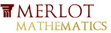# Effect of Coefficient of x^0 on Parabola VertexThis material was originally developed through Merlot
as part of its collaboration with the SERC Pedagogic Service.

## Summary

This classroom activity presents College Algebra students with a ConcepTest, a Question of the Day, and a Write-pair-share activity concerning the effect of the coefficient of x^0 (i.e., the constant, c) on the vertex of a parabola where a and b are arbitrarily fixed values in f(x)=ax^2+bx+c.

While students generally accept that the constant, c, produces a vertical shift in the graph of a parabola, most don't clearly understand why that happens. The project helps them to understand the nature of polynomial functions at a deeper level.

## Learning Goals

To enable students to:

• develop their understanding of the effects of coefficients in quadratic functions
• exercise their mathematical intuition and verify it via appropriate calculations
• exercise the skills of algebraic and mathematical analysis

## Context for Use

This activity can be carried out in either a small College Algebra class or a large lecture setting anytime during or after the topic of graphing quadratic functions has been covered.

The activity is comprised of four segments: ConcepTest, Question of the Day, Write-pair-share exercise, and conclusion involving the use of a demonstration applet. The time required for the entire activity is approximately 40 minutes but fewer segments can be offered as a shorter alternative (see Activity Description below for individual segment times).

## Description and Teaching Materials

Activity Description and Teaching Materials

• Instructor presents a ConcepTest (Rich Text File 55kB Aug18 10) in the form of a straw poll (either show-of-hands or written) concerning the effect of increasing the value of the coefficient of x^0 on the position of the vertex. Each student is asked to make a conjecture and the instructor records the results for the class to see. (~5 minutes)
• After the straw poll, the question becomes the Question of the Day (Rich Text File 53kB Aug18 10) and students work in pairs to share and explain their reasoning in written form. (~10 minutes)
• Lastly, students are asked to carry out the appropriate calculations in a write-pair-share activity (Rich Text File 117kB Aug18 10) to verify their conjectures. (~15 minutes)
• In conclusion, the instructor presents a classroom demo involving a Java applet that quickly and easily demonstrates the effects of changing the value of the coefficient of x^0 in a quadratic function. http://www.ltcconline.net/greenl/java/Other/MovingGraph/MovingGraph.html
In addition, the instructor ensures that students understand the algebraic and mathematical analysis involving the relationship between x and y and that increasing c while holding a and b fixed simply increases the value of y for any given value of x. (~10 minutes)

## Teaching Notes and Tips

Student understanding of the role of coefficients in polynomial functions is limited at best. Most have not considered the vertex in terms of the values of a, b and c, and many have difficulty in analyzing the effects of changing the values of these coefficients. Working with negative values can present a challenge. This activity is designed to help them develop a better grasp of the role of coefficients.

## Assessment

I generally give the students a grade for participation in this activity and sometimes grade their final calculations concerning the work required.

## References and Resources

Larry Green's Applet Page-Parameters and Graphs
This applet allows the user to change the values of the coefficients of a quadratic function and immediately see the graphical results. http://www.ltcconline.net/greenl/java/index.html
http://www.ltcconline.net/greenl/java/Other/MovingGraph/MovingGraph.html
MERLOT description of this resource
http://www.merlot.org/merlot/viewMaterial.htm?id=77279